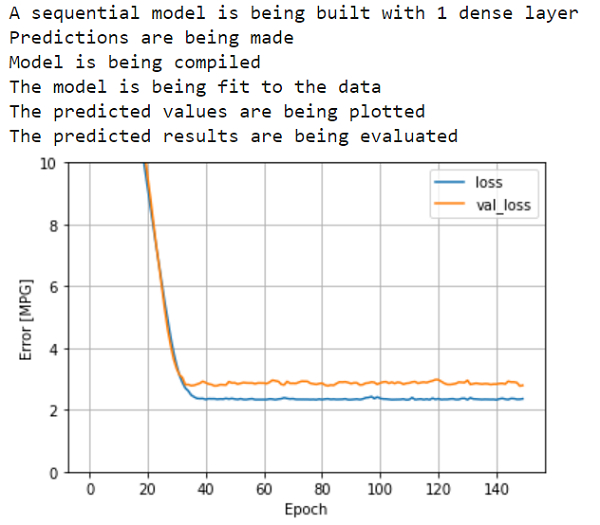# How can a sequential model be built on Auto MPG using TensorFlow?

PythonServer Side ProgrammingProgrammingTensorflow

Tensorflow is a machine learning framework that is provided by Google. It is an open−source framework used in conjunction with Python to implement algorithms, deep learning applications and much more. It is used in research and for production purposes. Tensor is a data structure used in TensorFlow. It helps connect edges in a flow diagram. This flow diagram is known as the ‘Data flow graph’. Tensors are nothing but multidimensional array or a list. They can be identified using three main attributes −

• Rank − It tells about the dimensionality of the tensor. It can be understood as the order of the tensor or the number of dimensions in the tensor that has been defined.

• Type − It tells about the data type associated with the elements of the Tensor. It can be a one dimensional, two dimensional or n dimensional tensor.

• Shape − It is the number of rows and columns together.

The ‘tensorflow’ package can be installed on Windows using the below line of code −

pip install tensorflow

The aim behind a regression problem is to predict the output of a continuous or discrete variable, such as a price, probability, whether it would rain or not and so on.

The dataset we use is called the ‘Auto MPG’ dataset. It contains fuel efficiency of 1970s and 1980s automobiles. It includes attributes like weight, horsepower, displacement, and so on. With this, we need to predict the fuel efficiency of specific vehicles.

A sequential model is a model that builds upon stack of layers.

We are using the Google Colaboratory to run the below code. Google Colab or Colaboratory helps run Python code over the browser and requires zero configuration and free access to GPUs (Graphical Processing Units). Colaboratory has been built on top of Jupyter Notebook. Following is the code snippet −

## Example

print("A sequential model is being built with 1 dense layer")
linear_model = tf.keras.Sequential([
normalizer,
layers.Dense(units=1)
])

linear_model.predict(train_features[:10])
linear_model.layers.kernel
print("Model is being compiled")
linear_model.compile(
loss='mean_absolute_error')
print("The model is being fit to the data")
history = linear_model.fit(
train_features, train_labels,
epochs=150,
verbose=0,
validation_split = 0.25)
print("The predicted values are being plotted")
plot_loss(history)
print("The predicted results are being evaluated")
test_results['linear_model'] = linear_model.evaluate(
test_features, test_labels, verbose=0)

## Output## Explanation

• A sequential architecture model is built using keras API.